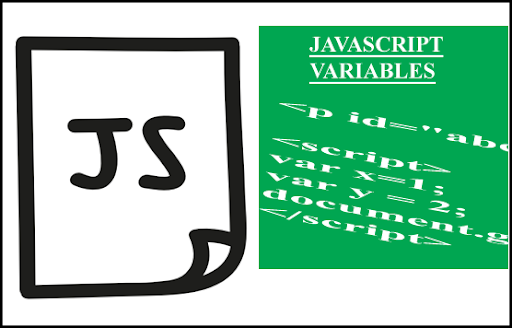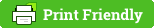# How to use Variables in the javascript?

Hi friends in this post I am sharing javascript variables .Declaring variables is one of the important step in javascript##### What is a variable?
Variable is used to store a value which can be used  when required.

Syntax of javascript variables
var x=1;

Example of javascript variable:

<p id="abc"></p>
<script>
var x=1;
var y = 2;
document.getElementById("abc").innerHTML = x+y;
</script>
Now you will get the output 3.
Note: We must declare semicolon at the end of variable name.

## What will happen when you use same variable multiple times in javascript?

Copy and paste the below code in notepad and save as index.html .Then open with the browser.

<p id="abc"></p>
<script>
var x=1;
var x = 2;
var x = 3;
var x = 4;
var x = 5;
document.getElementById("abc").innerHTML = x;
</script>
You will get the output 5 .

(i.e) Javascript will display the last value given in x ,if the same variable declared for multiple times.

## How to declare string in the javascript variables?

##### What is a string?
A string is a series of characters.For better understanding I can say it as words . Examples of string  are book,bag,vegetables.
String must be placed in between the single or double quotes . It’s your wish to use single or double quotes.

Example to describe how to declare string in javascript:

<p id="abc"></p>
<script>
var bookname="English";
document.getElementById("abc").innerHTML = bookname;
</script>
Here English is the string. bookname is the variable.
##### What will happen if we not using quotes in string?
Very simple.There will be no output .

## What are the effects of with and without using quotes ?

Consider the below example ,

<p id="abc"></p>
<script>
var x='1';
var y = '2';
document.getElementById("abc").innerHTML = x+y;
</script>

Copy and paste the above code in notepad and save as index.html .Then open with the browser.
You will get the output 12 and not 3 . Because after using the quotes the values are considered as string and + symbol use the concatenate process like adding strings .

Also try the below example ,

<p id="abc"></p>
<script>
var x="english";
var y = "tamil";
document.getElementById("abc").innerHTML = "there are " +x+y+  " books"  ;
</script>
You will get output as there are englishtamil books .

Another example:

<p id="abc"></p>
<script>
var x=8;
var y = 9;
document.getElementById("abc").innerHTML = "there are " +x+y+  " books";
</script>
Note: Here I have used variable name values as numbers and not as string. But it will show 89 instead of 17
because x is concatenated with the string there are .